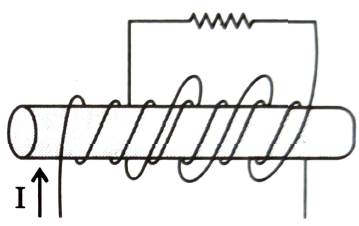Magnetic flux and induced emf
Question

# A coil with 1500 turns, a radius of 5.0 cm and a resistance of 12$\mathrm{\Omega }$ surrounds a solenoid with 250 turns/cm and a radius of 4 cm; see figure. The current in the solenoid changes at a constant rate from 0 to 20 A in 0.10 s. The magnitude of the induced current (in A) in the 1500 turn coil is ( Take ${\pi }^{2}=10$,  Neglect self inductance of the coil)Moderate
Solution
Get Instant Solutions